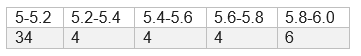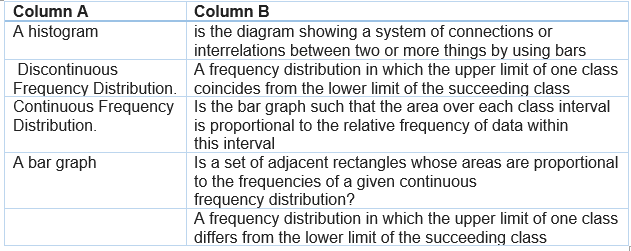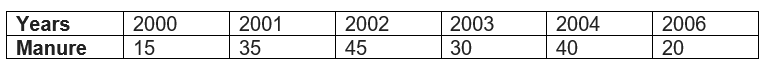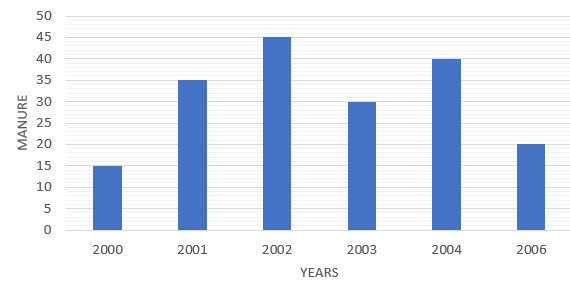# Statistics class 9 extra questions

Given below are the Class 9 Maths Important Questions for statistics
a. Concepts questions
b. Calculation problems
c. Multiple choice questions
d. Match the Column
e. Fill in the blank's

## Fill in the blank

(a) The mean of the data set (4 , 10 , 7 , 7 , 6 , 9 , 3 , 8 , 9 )  is ___________ (7/8/9)
(b) The median of the above data set is _______ (7/8/9)
(c) There are ______ Modes of the above given data set (1/2/3)
(d) The range of the data set (78 , 65 , 68 , 72 , 70 , 76 , 74 , 62 , 80 , 82 , 96 , 101) is _______ (56/39/40)
(e) The mean of five numbers is 40. If one number is excluded, their mean becomes 28.The excluded number is _____ (68/88)
(f) The class size of the grouped size frequency table given below is__________ (.1/.2)

## True or False statement

Question 2
Two sections of Class XII having 30 students each appeared for Science Olympiad. The marks obtained by them are shown below:
46 31 74 68 42 54 14 61 83 48 37 26 8 64 57
93 72 53 59 38 16 88 75 56 46 66 45 61 54 27
27 44 63 58 43 81 64 67 36 49 50 76 38 47 55
77 62 53 40 71 60 58 45 42 34 46 40 59 42 29
Student having Marks above 80 are exceptional
Student obtaining below 30 marks are failed
(a) The no of student who scored more than 89 marks is 2
(b) The number who scored, marks between 50-69 is 22
(c) The number of student who scored more than 49 marks is 32
(d) The range of the marks is 85
(e) The no of exceptional students are 4
(f) Student who failed in the test are 7

## Multiple choice Questions

Question 3
Find x and y so that the ordered data set has a mean of 42 and a median of 35.
17, 22, 26, 29, 34, x, 42, 67, 70, y
(a) x=34, y=77
(b) x=36, y=77
(c) x=77, y=34
(d) x=77, y=36
Solution
(b)

Question 4
For what value of n, the mode of the following data is 18?
2, 5, 3, 18, 5, 18, 6, 5, n, 7, 18
(a) 18
(b) 5
(c) It can be any value
(d) None of these
Solution
(a)
There are three 5 and three 18.
For mode to be 18, n=18

Question 5
For drawing a frequency polygon of a continuous frequency distribution, we plot the Points whose ordinates are the frequencies of the respective classes and abscissae are respectively:
(a) Class marks of the classes
(b) Upper limits of preceding classes
(c) Lower limits of the classes
(d) Upper limits of the classes
Solution
(a)

Question 6
There are 150 numbers. Each number is subtracted from 60 and the mean of the numbers so obtained is found to be –4.5. The mean of the given numbers is
(a) 400
(b) 34.5
(c) 64.5
(d) 55.5
Solution
(d)
Mean of number -60=-4.5
So mean of number is 55.5

Question 7
The median and mean of the first 10 natural numbers are,
(a) 5.5,5.5
(b) 5.5,6
(c) 5,6
(d) None of these
Solution
(a)
Mean =5.5
Median is  mean of 5 and 6 th term, So 5

Question 8
Anand says that the median of 3, 14, 19, 20, 11 is 19. What doesn’t the Anand understand about finding the median?
(a) The dataset should be ascending order
(b) Highest no in the dataset is the median
(c) Average of lowest and highest is the median
(d) None of these
Solution
(a)

Question 9
The following observations are arranged in ascending order :
20, 23, 42, 53, x, x + 2, 70, 75, 82, 96
If the median is 63, find the value of x.
(a) 62
(b) 64
(c) 60
(d) None of these
Solution
(a)
Median is mean of 5 and 6 term
So x+1=63
x=62

Question 10
The mean of 20 observations was 60. It was detected on rechecking that the value of 125 was wrongly copied as 25 for computation of mean. Find the correct mean
(a) 67
(b) 66
(c) 65
(d) None of the above
Solution
(c)
Let x be the sum of observation of 19 numbers leaving 125,
Then
$x+25=20 \times 60=1200$
Now
$x+125=20 \times y=20y$
Subtracting
$125-25=20y-1200$
$20y=1300$
$y=65$

## Match the column

Question 11Subjective Questions Question 12
The following data gives the amount of manure (in tones) manufactures by a company during some years.1. Represent it with a bar graph.
2. Indicate with help of bar graph in which year, the amount of manufactured by company was maximum.
SolutionQuestion 13
The following observations are arranged in ascending order :
26, 29, 42, 53, x, x + 2, 70, 75, 82, 93
If the median is 65, find the value of x
Solution
Number of observations (n) = 10, which is even. Therefore, median is the mean of the (n/2) and (n/2 +1) terms
i.e., 5th and 6th observation.
Here, 5th observation = x
6th observation = x + 2
Therefore Median is
$\frac {x + x +2}{2}=65$
Therefore, x = 64

Question 14
Mean of 50 observations was found to be 80.4. But later on, it was discovered that 96 was misread as 69 at one place. Find the correct mean
Solution
Mean is given by
$M= \frac {c}{n}$
Therefore
$\sum_{1}^{n} x_i =nM$
Here $\sum_{1}^{n} x_i =50 \times 80.4$
Now After the mistake was discovered
$\sum_{1}^{n} x_i =50 \times 80.4 -96 + 69=3993$
So correct mean will be
$M_c= 3993/50=79.86$

## Summary

This statistics class 9 extra questions with answers is prepared keeping in mind the latest syllabus of CBSE . This has been designed in a way to improve the academic performance of the students. If you find mistakes , please do provide the feedback on the mail.